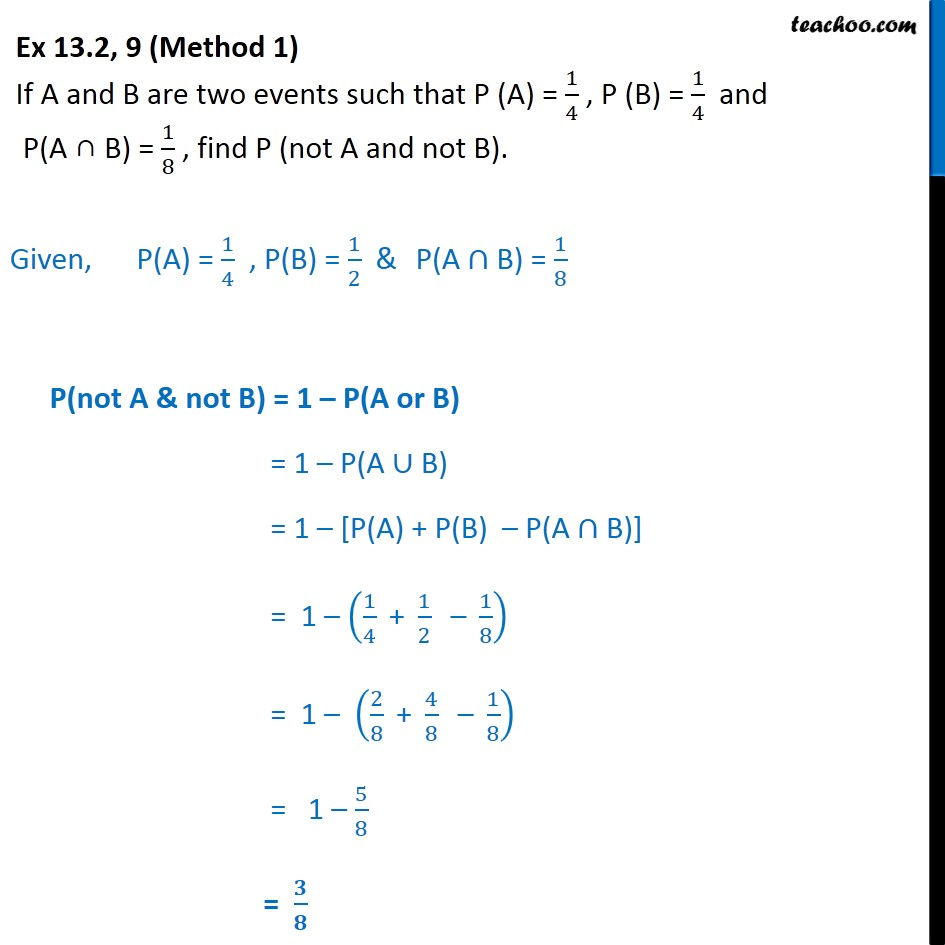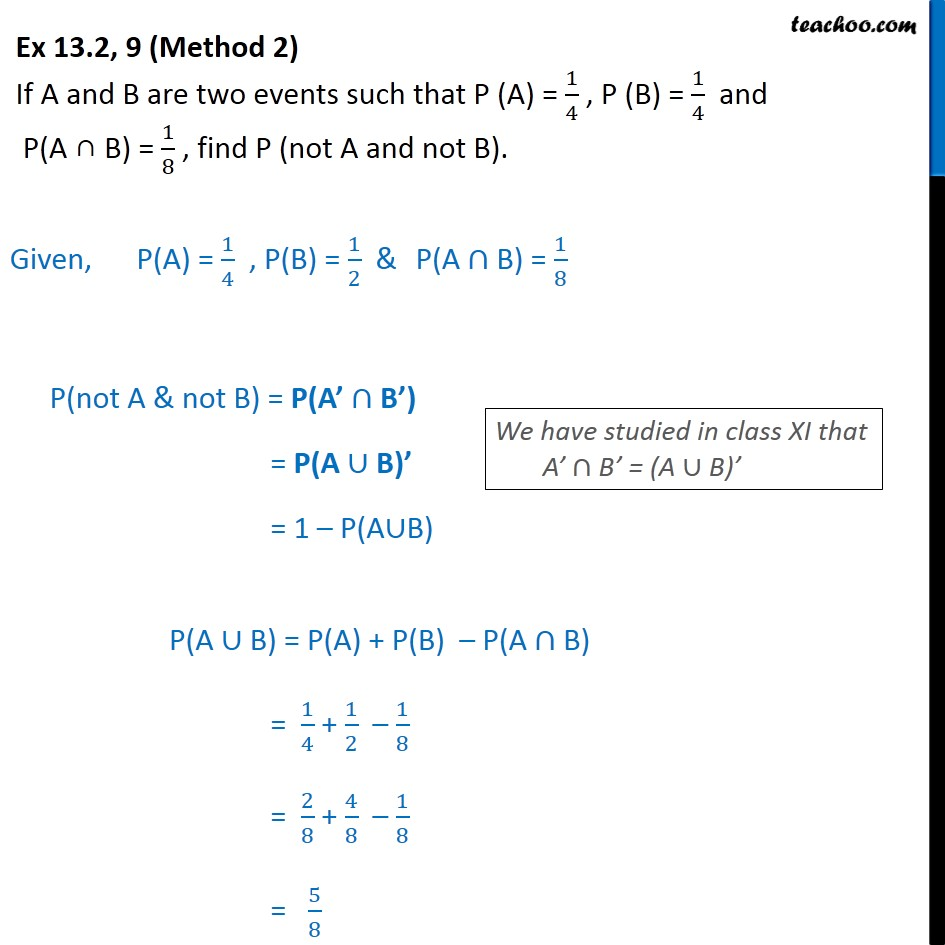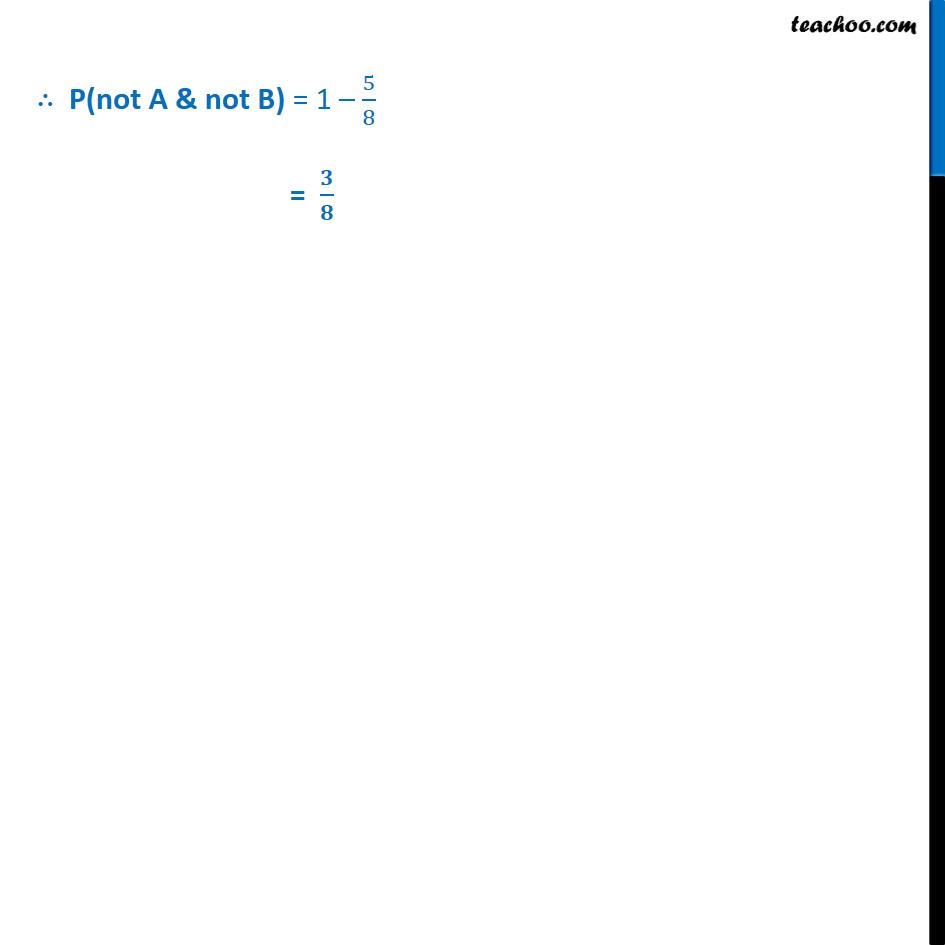1. Chapter 13 Class 12 Probability
2. Serial order wise
3. Ex 13.2

Transcript

Ex 13.2, 9 (Method 1) If A and B are two events such that P (A) = 1﷮4﷯ , P (B) = 1﷮4﷯ and P(A ∩ B) = 1﷮8﷯ , find P (not A and not B). Given, P(A) = 1﷮4﷯ , P(B) = 1﷮2﷯ & P(A ∩ B) = 1﷮8﷯ P(not A & not B) = 1 – P(A or B) = 1 – P(A ∪ B) = 1 – [P(A) + P(B) – P(A ∩ B)] = 1 – 1﷮4﷯ + 1﷮2﷯ – 1﷮8﷯﷯ = 1 – 2﷮8﷯ + 4﷮8﷯ – 1﷮8﷯﷯ = 1 – 5﷮8﷯ = 𝟑﷮𝟖﷯ Ex 13.2, 9 (Method 2) If A and B are two events such that P (A) = 1﷮4﷯ , P (B) = 1﷮4﷯ and P(A ∩ B) = 1﷮8﷯ , find P (not A and not B). Given, P(A) = 1﷮4﷯ , P(B) = 1﷮2﷯ & P(A ∩ B) = 1﷮8﷯ P(not A & not B) = P(A’ ∩ B’) = P(A ∪ B)’ = 1 – P(A∪B) P(A ∪ B) = P(A) + P(B) – P(A ∩ B) = 1﷮4﷯ + 1﷮2﷯ – 1﷮8﷯ = 2﷮8﷯ + 4﷮8﷯ – 1﷮8﷯ = 5﷮8﷯ ∴ P(not A & not B) = 1 – 5﷮8﷯ = 𝟑﷮𝟖﷯

Ex 13.2